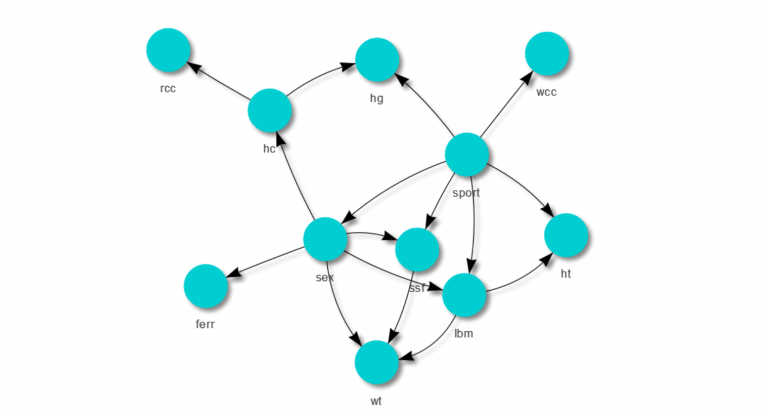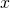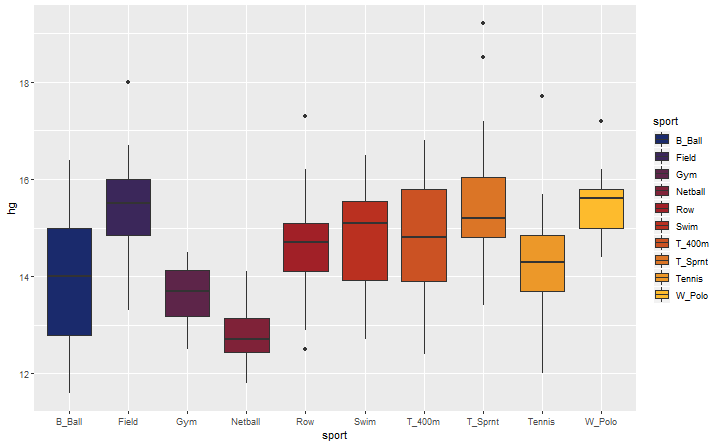Want to share your content on R-bloggers? click here if you have a blog, or here if you don't.Bayesian Networks are probabilistic graphical models and they have some neat features which make them very useful for many problems. They are structured in a way which allows you to calculate the conditional probability of an event given the evidence. The graphical representation makes it easy to understand the relationships between the variables and they are used in many AI solutions where decisions need to be automated in a range of contexts such as medical diagnosis, risk modelling and mitigation. Bayesian networks are great where the is a complex system of many causal relationships.

Some key benefits of Bayesian Networks include:

• It is easy to visualise the casual relationships and variable independence by the graphical representation.
• All parameters are interpretable.
• The model can be easily queried to calculate any conditional probability
• Predictions can be made about any variable, rather than there being a distinction between a dependentvariable and explantoryvariables such as in a regression model.
• Can easily handle missing or sparse data.
• Relationships can be chained which allows for more complex inference and scalability.

In this post I’ll build a Bayesian Network with the AIS dataset found in the DAAG package. This dataset was used to determine if there was a difference in mean hemoglobin levels for different sport disciplines. To begin with we’ll quickly look at a box plot comparing the distribution of hemoglobin levels for the different sports just to get a feel for the data.

`ggplot(ais, aes(x = sport, y = hg, fill = sport)) + geom_boxplot() + scale_fill_manual(values = colorRampPalette(king.yna)(10))`The box plots would suggest there are some differences. We can use this to direct our Bayesian Network construction.

### Discrete case

We’ll start of by building a simple network using 3 variables hematocrit (hc) which is the volume percentage of red blood cells in the blood, sport and hemoglobin concentration (hg). Hematocrit and hemoglobin measurements are continuous variables. For simplicity of the first example these will be transformed to binary variables and we’ll subset the data to only 3 sports, netball, tennis and water polo. These sports were chosen since there is a clear difference between their hemoglobin levels as shown by the box plots above. An empty graph will be created followed by inputting the structure manually.

```# set boolean variables
ais\$high_hc <- as.factor(ais\$hc > median(ais\$hc))
ais\$high_hg <- as.factor(ais\$hg > median(ais\$hg))

# create an empty graph
structure <- empty.graph(c("high_hc", "high_hg", "sport"))

# set relationships manually
modelstring(structure) <- "[high_hc][sport][high_hg|sport:high_hc]"

# plot network func
# using the visNetwork package to plot the network because it looks very nice.
plot.network <- function(structure, ht = "400px"){
nodes.uniq <- unique(c(structure\$arcs[,1], structure\$arcs[,2]))
nodes <- data.frame(id = nodes.uniq,
label = nodes.uniq,
color = "darkturquoise",

edges <- data.frame(from = structure\$arcs[,1],
to = structure\$arcs[,2],
arrows = "to",
smooth = TRUE,
color = "black")

return(visNetwork(nodes, edges, height = ht, width = "100%"))
}

# observe structure
plot.network(structure)```

If you can’t see the plot you may need to unblock the content or see it here. There are algorithms to do this which I’ll briefly go into later, but for now we’ll explicitly state the relationships. Manually creating the structure is often a good way to go since you are required to understand the system you are trying to model and not relying on a black box to do it for you. Having said that, once constructed whether it be manually or using an algorithm the Bayesian Network is easily understood through the graphical representation and each variable can be explained.

The relationship of this network is saying,

• Hemoglobin levels are conditionally dependent on hematocrit levels and the sport
• Sport and hematocrit levels are independent.

We’ll now fit the model and output the conditional probabilities for each node.

```ais.sub <- ais[ais\$sport %in% c("Netball", "Tennis", "W_Polo"), c("high_hc", "high_hg", "sport")]
ais.sub\$sport <- factor(ais.sub\$sport)
bn.mod <- bn.fit(structure, data = ais.sub)
bn.mod```

```##
##   Bayesian network parameters
##
##   Parameters of node high_hc (multinomial distribution)
##
## Conditional probability table:
##      FALSE      TRUE
## 0.6078431 0.3921569
##
##   Parameters of node high_hg (multinomial distribution)
##
## Conditional probability table:
##
## , , sport = Netball
##
##        high_hc
## high_hg     FALSE TRUE
##   FALSE 1.0000000
##   TRUE  0.0000000
##
## , , sport = Tennis
##
##        high_hc
## high_hg     FALSE      TRUE
##   FALSE 0.8571429 0.2500000
##   TRUE  0.1428571 0.7500000
##
## , , sport = W_Polo
##
##        high_hc
## high_hg     FALSE      TRUE
##   FALSE 1.0000000 0.0625000
##   TRUE  0.0000000 0.9375000
##
##
##   Parameters of node sport (multinomial distribution)
##
## Conditional probability table:
##    Netball    Tennis    W_Polo
## 0.4509804 0.2156863 0.3333333```

`cat("P(high hemaglobin levels) =", cpquery(bn.mod, (high_hg=="TRUE"), TRUE), "\n")`

`## P(high hemaglobin levels) = 0.2136`

`cat("P(high hemaglobin levels | play water polo and have high hematocrit ratio) =", cpquery(bn.mod, (high_hg=="TRUE"), (sport == "W_Polo" & high_hc == "TRUE")), "\n")`

`## P(high hemaglobin levels | play water polo and have high hematocrit ratio) = 0.9399076`

One of the main benefits of Bayes nets is we can reverse the direction. Unlike a regression where there are response and explanatory variables a Bayes Net is not ‘fixed’ (for lack of a better word) in the same way and each node can be made the subject of the query for inference. With the same model we can query the probability that an athlete plays water polo given we observe their high hemoglobin levels or the probability of having high hemoglobin levels given they play water polo.

`cat("P(they play water polo | high hemaglobin levels and have high hematocrit ratio) =", cpquery(bn.mod, (sport=="W_Polo"), (high_hg == "TRUE" & high_hc == "TRUE")), "\n")`

`## P(they play water polo | high hemaglobin levels and have high hematocrit ratio) = 0.6351064`

Let’s say that we didn’t know the athletes hematocrit ratio, could we still calculate the probability they have high hemoglobin levels given they play water polo? Sure, we just sum over the hematocrit probability distribution. Fortunately the `cpquery` function takes care of this for us.

`cat("P(high hemaglobin levels | play water polo) =", cpquery(bn.mod, (high_hg=="TRUE"), (sport == "W_Polo")), "\n")`

`## P(high hemaglobin levels | play water polo) = 0.3623018`

### Continuous case

Let’s redefine our simple network with the actual continuous variables. Again, `bnlearn` handles the hard work. For the continuous case the probability densities are estimated.

```# create an empty graph
structure <- empty.graph(c("hc", "hg", "sport"))

# set relationships manually
modelstring(structure) <- "[hc][sport][hg|sport:hc]"

# subset and fit
ais.sub <- ais[ais\$sport %in% c("Netball", "Tennis", "W_Polo"), c("hc", "hg", "sport")]
ais.sub\$sport <- factor(ais.sub\$sport)
bn.mod <- bn.fit(structure, data = ais.sub)
bn.mod```

```##
##   Bayesian network parameters
##
##   Parameters of node hc (Gaussian distribution)
##
## Conditional density: hc
## Coefficients:
## (Intercept)
##    41.82353
## Standard deviation of the residuals: 4.092363
##
##   Parameters of node hg (conditional Gaussian distribution)
##
## Conditional density: hg | hc + sport
## Coefficients:
##                       0           1           2
## (Intercept)   1.5550754  -2.7611358  -0.1173597
## hc            0.2929909   0.4019544   0.3398915
## Standard deviation of the residuals:
##         0          1          2
## 0.2726074  0.3383277  0.3091150
## Discrete parents' configurations:
##      sport
## 0  Netball
## 1   Tennis
## 2   W_Polo
##
##   Parameters of node sport (multinomial distribution)
##
## Conditional probability table:
##    Netball    Tennis    W_Polo
## 0.4509804 0.2156863 0.3333333```

Now when querying the model we need to be a little more specific than in the discrete case by specifying a range.

`cat("P(hemaglobin levels > 14 | play water polo and have high hematocrit ratio) =", cpquery(bn.mod, (hg > 14), (sport == "W_Polo" & hc > 42 )), "\n")`

`## P(hemaglobin levels > 14 | play water polo and have high hematocrit ratio) = 0.9495798`

### Chained relationships

Another key benefit of Bayes nets is variables can be chained together. In other words two nodes don’t need to be directly connected to make inference from one about the other. We’ll add in another variable into our simple model, lean body mass which is calculated as body weight minus body fat in kgs, so higher the number the leaner the athlete.

```# create an empty graph
structure <- empty.graph(c("hc", "hg", "sport", "lbm"))

# set relationships manually
modelstring(structure) <- "[lbm][hc|lbm][sport][hg|sport:hc]"
plot.network(structure)

# subset and fit
ais.sub <- ais[ais\$sport %in% c("Netball", "Tennis", "W_Polo"), c("hc", "hg", "sport", "lbm")]
ais.sub\$sport <- factor(ais.sub\$sport)
bn.mod <- bn.fit(structure, data = ais.sub)
bn.mod```

```##
##   Bayesian network parameters
##
##   Parameters of node hc (Gaussian distribution)
##
## Conditional density: hc | lbm
## Coefficients:
## (Intercept)          lbm
##  26.5212185    0.2471436
## Standard deviation of the residuals: 2.846647
##
##   Parameters of node hg (conditional Gaussian distribution)
##
## Conditional density: hg | hc + sport
## Coefficients:
##                       0           1           2
## (Intercept)   1.5550754  -2.7611358  -0.1173597
## hc            0.2929909   0.4019544   0.3398915
## Standard deviation of the residuals:
##         0          1          2
## 0.2726074  0.3383277  0.3091150
## Discrete parents' configurations:
##      sport
## 0  Netball
## 1   Tennis
## 2   W_Polo
##
##   Parameters of node sport (multinomial distribution)
##
## Conditional probability table:
##    Netball    Tennis    W_Polo
## 0.4509804 0.2156863 0.3333333
##
##   Parameters of node lbm (Gaussian distribution)
##
## Conditional density: lbm
## Coefficients:
## (Intercept)
##    61.91667
## Standard deviation of the residuals: 12.00722```

Network plot

Now we can query the model and calculate the probability that athletes have hemoglobin levels greater than 14 given they play water polo and have an LBM of greater than 65 kg without having any knowledge of their hematocrit ratio.

`cat("P(hemaglobin levels > 14 | play water polo and have LBM > 65 kg) =", cpquery(bn.mod, (hg > 14), (sport == "W_Polo" & lbm > 65 )), "\n")`

`## P(hemaglobin levels > 14 | play water polo and have LBM > 65 kg) = 0.8123028`

### Algorithmically defined structure

For large cases you’ll want to use an algorithm to define the structure of the Bayes net, and then add other user defined relationships on top of that if required. `bnlearn` includes the hill climbing algorithm which is suitable for the job. The default score it uses to optimise the model is the BIC which is appropriate. There are many others such as AIC, Bayesian Dirichlet score, K2, to name a few that may be more appropriate for your problem.

```# learn the structure using the hill climbing algorithm and the BIC
structure <- hc(ais.sub, score = "bic-cg")
plot.network(structure)```

Network plot

As you can see it is different to the one defined. This structure best fits the data by maximising the BIC, but if we understand the system well enough we can input the relationships that we know are important. This is more the case when sample sizes are small, when they are large we can put more trust in the algorithm to find the correct relationships. Having said that, often there are biases in the data and if those mechanisms are well understood the right relationships can be put into the model as well.

```bn.mod <- bn.fit(structure, data = ais.sub)
cat("P(hemaglobin levels > 14 | play water polo and have LBM > 65 kg) =", cpquery(bn.mod, (hg > 14), (sport == "W_Polo" & lbm > 65 )), "\n")```

`## P(hemaglobin levels > 14 | play water polo and have LBM > 65 kg) = 0.9833866`

### Full model

Now we will fit the full model using all the available data after removing those which are a function of others e.g..

```ais.sub <- ais[, c("hc", "hg", "sport", "lbm", "rcc", "wcc", "ferr", "ht", "wt", "sex", "ssf")]
structure <- hc(ais.sub, score = "bic-cg")
bn.mod <- bn.fit(structure, data = ais.sub)
plot.network(structure, ht = "600px")```

Network plot

Bayes Nets can get complex quite quickly (for example check out a few from the bnlearn doco, however the graphical representation makes it easy to visualise the relationships and the package makes it easy to query the graph.

### Using the output

Fitting the network and querying the model is only the first part of the practice. Where Bayes nets really shine is how they are used to make actionable decisions. In our example we fit a model to help explain the influencing factors on hemoglobin concentration in an athlete. But lets assume that high hemoglobin levels are correlated with better performance, which is likely to be true for endurance sports such as running or cycling but less so for skill based sports like basketball. The athlete could take appropriate action to ensure their hemoglobin concentrations are at optimal levels. Decisions need to be made around

• Diet – What to eat on certain days e.g. training days versus taper days
• Training – When to increase or decrease the intensity and frequency
• Rest – When to take a rest day or recover after a game/race
• Illness – How long do you need to recover?

It is when ‘interventions’ such as these can be accounted for in the model the user can implement ‘what if’ scenarios to help make the best decision. Some of these variables can easily be observed but other can not such as red cell count. This might be a measurement that gets taken once every 2-3 months perhaps, in which case decisions will need to be made without the knowledge of the athletes current red cell count. Fortunately a Bayesian network can handle this type of uncertainty and missing information.

The outputs of a Bayesian network are conditional probabilities. Often these are used as input for an overarching optimisation problem. For example an insurance company may construct a Bayesian network to predict the probability of signing up a new customer to premium plan for the next marketing campaign. This probability is then used to calculate the expected revenue from new sales. In turn the model could inform the company if they took actions A and B they could increase their revenue by \$x or if they advertised in these other locations for some cost, the revenue is expected to be \$y. Using this information they can make them best decision to maximise their profits.

Bayesian networks are hugely flexible and extension to the theory is a Dynamic Bayesian Network which brings in a time component. As new data is collected it is added to the model and the probabilities are updated. This is homework for another day.

The post Bayesian Network Example with the bnlearn Package appeared first on Daniel Oehm | Gradient Descending.